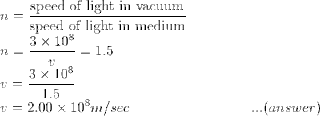## Pages

Showing posts with label class10-physics-numericals. Show all posts
Showing posts with label class10-physics-numericals. Show all posts

## CBSE Class 10 - Physics - Study Notes - MCQs, Worksheets, NCERT Solutions

Physics is a pivotal subject for students who wish to seek a career in engineering and other related fields. The majority of the ideas in class 10 Physics are significant as they build the foundations for senior-level physics that are taught in class eleventh and twelfth. Along these lines, having an intensive comprehension of the considerable number of ideas is pivotal to get higher marks in school tests as well as get acquainted with wide a range of information.

In this way, to assist the students with learning the subject more viably, physics notes for class 10 students are given here. These notes based on CBSE syllabus are all around organized, straightforward and spread all the significant points that students need to learn for the tests.

In addition, students can utilize the notes to not just ace in their assessment tests but are effecting in learning the important concepts. There are also 1 mark questions, MCQs and worksheets that will help students evaluate their knowledge about various topics. The notes are written in straightforward language and help students grasp basic concepts easily.

You can check out links to the study notes on various topics, exercises and chapters related to class 10 Physics are given below:## Sunday, 1 April 2012

### Class 10 - Science - Ch12 - Electricity - Q & A

Electricity

Q & A

Q1: What type of charge does an ebonite rod acquire when it is rubbed with wool? What is
the nature of the charge acquired by wool?

Answer: Ebonite rod has a negative charge and woollen cap has an equal amount of positive charge.

Note: Charging by friction always produces equal and opposite charges on the twobodies which are rubbed in intimate contact.

Q2: What is the charge on an electron (e)?

Answer: -1.6 x 10 -19 Coulombs (C)

Q3: Define Coulomb's law.

Answer: The magnitude of the force of attraction (or repulsion) between two point charges is
directly proportional to the quantity of charge present on each of them and inversely
proportional to the square of the distance separating them.

Let q1 and q2 be two like charges separated by a distance d, then the two charges will continue to repel each other with a force (F). Mathematically it is represented as:

Q4: What is the SI unit of charge? Is it a scalar or vector quantity?
Answer: SI unit of charge is Coulomb (C). It is a scalar quantity.## Class 10 - Physics - Light - Numerical and Questions

Q1: When an object is placed at a distance of 60 cm from a convex mirror, the magnification produced is 1/2.
Where should the object be placed to get a magnification of 1/3?

Q2: When an object is kept at a distance of 60cm from a concave mirror, the magnification is 1/2. Where should the object be placed to get a magnification of 1/3?

Answer: (Hint: steps are the same as in the above question, except it is a concave mirror, m = -1/2. Compute f in the first case i.e. f = -20 cm and the compute u in case II i.e. u = 80 cm)

Q3(CBSE Board): A concave lens made of material of refractive index (n1) is kept in a medium of refractive index (n2). A parallel beam of light is incident on the lens. Complete the path of the rays of light emerging from a concave lens if:
(a) n1 > n2
(b) n1 = n2
(c) n1 < n2## Numerical Problem on Light Reflection and Refraction

Q1: How fast does the light travel in a glass of refractive index 1.5?

By Snell's law, refractive index (n) is the ratio of i.e.

Q2:When a bright object is placed 10 cm away from a concave mirror, its real image is formed at a distance 40cm from the mirror. What is the focal length of the mirror?Q3: If the angle of incidence of a ray of light falling on the glass surface is 30° and the angle of refraction is 19°. what is the refractive index of glass?

Ans:
angle of incidence  i = 30°
angle of refraction  r = 19°
refractive index   n = sin i/sin r  = sin 30/sin 19
n = .5/.3256 = 1.535               ....(ans)

Q4: If a child crawls towards a mirror at a rate of 0.20 m/s, then at what speed will the child and her image come nearby to each other?

Ans: In 1 sec, the child moves towards the mirror by a distance of 0.20 meters. In the same second, her image also moves 0.20m closer.
Therefore, the child and her image comes closer to each other by 0.40m per second.